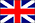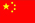Hi, welcome to Dongguan Haoda Electronic Co., Ltd.!
EnglishChineseproduct center
4dhcpmingchenMagnetic ring inductance coils
The magnetic ring inductance coil is mainly used for the switch power supply. For manufacturing the filter inductor (inductance coil) and transformer, the electromagnetic inductance elements wound with insulating conductors (such as enameled wire, cotton covered wire and so on). Such coil is also the one of common components of the electronic circuit. The relevant products include common mode filter and so on. It has such characteristics as low consumption, high magnetic conductivity, low cost and so on.
I. Self-inductance and mutual inductance
(1) Mutual inductance
When the current passes through the coil, there will be magnetic field around the coil. When the current in the coil changes, the magnetic field around the coil will also change, the changing magnetic field can make the coil itself to have induction electromotive force (the induction electromotive force is used to express the terminal voltage of the ideal power supply of the active element), this is self inductance.
(2) Mutual inductance
When two inductance coils mutually approaches, change of the magnetic field of an inductance coil will affect another inductance coil, this influence is just mutual inductance. Size of mutual inductance depends on the coupling degree between the self-inductance of the inductance oil and two inductance coils.
If one capacitance is connected to the DC power supply, there will be charging at the time when the power supply is switched on. Because Q=CU, and there will instantaneous directional movement of charge in the circuit and short-time current. However, after it is stable, the charge in the circuit will not move, and there will not be current in the circuit. When the circuit changes, the voltages at both ends of the capacitance will reduce, the capacitance will discharge. Because Q=CU, after it is stable, there will not be function of current in the circuit.
Because the voltage of AC power supply continuously changes, and thus the capacitance will continuously charge. Thus, the current seems to pass through the capacitance, but the fact is not so.
Of course, the capacitance also has impedance effect on AC power supply.
Inductance is energy-saving element, is mainly used for the power supply filter circuit and focuses on inhibiting conductive disturbance; the magnetic bead is mainly used for signal circuit and mainly used for EMI. In PCB board circuit of the electronic equipment, a great deal of inductive elements and EMI filter elements will be used. These elements include chip type inductance and dry type magnetic bead. In the following characteristics of these two elements will be described, and their ordinary application conditions and special application conditions will be analyzed. SMD element has the advantages that it has small packaging size and can meet requirement of the actual space. Except the impedance value, current carrying capacity and other similar physical characteristics, other performance characteristics of the go-through hole connector and SMD element are basically same. For the condition where the chip inductance is used, the inductance is required to have the following two basic functions: circuit resonance and choking reactance. Resonance circuits include the resonance generating circuit, oscillating circuit, clock circuit, impulse circuit, waveform generating circuit and so on. The resonance circuit also includes high Q band pass filter circuit. For producing circuit resonance, there must be capacitance and inductance in the circuit at the same time. There is parasitic capacitance at both ends of the inductance because the ferrite between two electrodes is equivalent to the capacitance dielectric material. In resonance circuit, the inductance must have high Q, narrow inductance deviation and stable temperature coefficient in order to meet the resonance circuit’s requirements (narrow band and low frequency temperature drift). High Q circuit has the sharp resonance peak value. Narrow inductance deviation can guarantee the minimum resonance frequency deviation. Stable temperature coefficient can guarantee that the resonance frequency has stable temperature change characteristic. Difference among the standard the radial inductive inductance, axial inductive inductance and chip inductance only is the different packaging. The inductance structure consists of the coil wound on the dielectric material (usually, alumina ceramic material), or the hollow coil or the coil wound on the ferromagnetism material. For power application conditions, if used as the choking coil, the main parameters of the inductance are DC resistance (DCR), rated current and low Q value. If used as the filter, wide band width characteristic is desired. Thus, it does not need high Q characteristic of the inductance. Low DCR can guarantee the minimum voltage drop. DCR means the DCR when the element does not have AC signal.